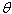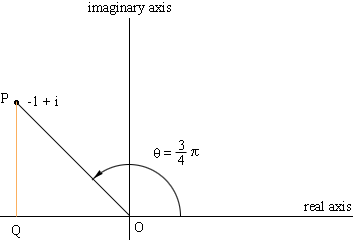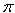SEARCH HOMEMath Central Quandaries & QueriesSubject: Exponential form Name: Austin Who are you: Student When dealing with imaginary numbers in engineering, I am having trouble getting things into the exponential form. The equation is -1+i now I do know that re^(theta)i = r*cos(theta) + r*i*sin(theta). Just not quite understanding the order of operations. ThanksHi Austin,

To express -1 + i in the form r ei= r (cos() + i sin()) I think of the geometry. In the complex plane plot the point -1 + i.The modulus r of p = -i + i is the distance from O to P. Since PQO is a right triangle Pythagoras theorem tells you that r = √2. The argument of P is the angle,measured counterclockwise from the positive real axis to the line segment OP. Here= 3/4. Cos( 3/4) = -1/√2 and sin(3/4) = 1/√2. Thus

You don't need to use the diagram. The modulus of x + iy is √(x2 + y2) which for -i + i is

r = √((-1)2 + 12) = √2

Also x = r cos () and y = r sin() so -1 = √2 cos () and 1 = √2 sin (). This gives= 3/4.

PennyMath Central is supported by the University of Regina and The Pacific Institute for the Mathematical Sciences.